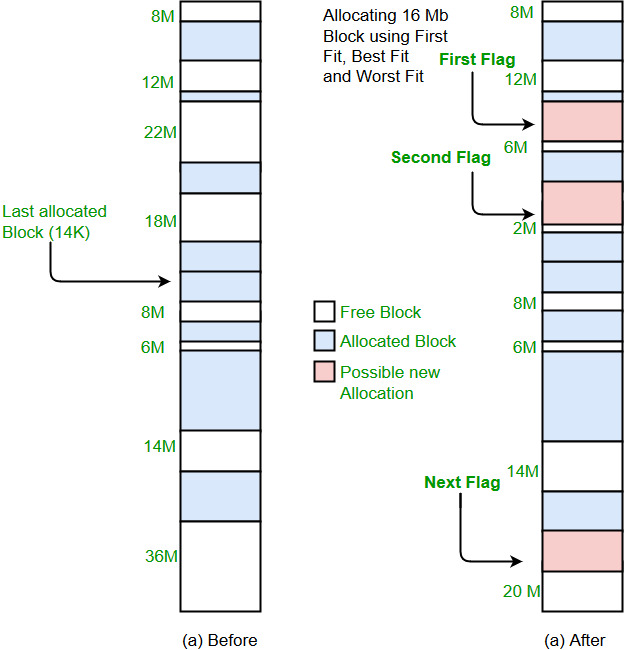Related Articles

# Program for First Fit algorithm in Memory Management

• Difficulty Level : Basic
• Last Updated : 28 Jun, 2021

Prerequisite : Partition Allocation Methods

In the first fit, the partition is allocated which is first sufficient from the top of Main Memory.

Example :

```Input : blockSize[]   = {100, 500, 200, 300, 600};
processSize[] = {212, 417, 112, 426};
Output:
Process No.    Process Size    Block no.
1               212            2
2               417            5
3               112            2
4               426        Not Allocated
```
• Its advantage is that it is the fastest search as it searches only the first block i.e. enough to assign a process.
• It may have problems of not allowing processes to take space even if it was possible to allocate. Consider the above example, process number 4 (of size 426) does not get memory. However it was possible to allocate memory if we had allocated using best fit allocation [block number 4 (of size 300) to process 1, block number 2 to process 2, block number 3 to process 3 and block number 5 to process 4].

## Recommended: Please try your approach on {IDE} first, before moving on to the solution.

```Implementation:
1- Input memory blocks with size and processes with size.
2- Initialize all memory blocks as free.
3- Start by picking each process and check if it can
be assigned to current block.
4- If size-of-process <= size-of-block if yes then
assign and check for next process.
5- If not then keep checking the further blocks.
```Below is an implementation of above steps.

## C++

 `// C++ implementation of First - Fit algorithm``#include``using` `namespace` `std;`` ` `// Function to allocate memory to ``// blocks as per First fit algorithm``void` `firstFit(``int` `blockSize[], ``int` `m, ``              ``int` `processSize[], ``int` `n)``{``    ``// Stores block id of the ``    ``// block allocated to a process``    ``int` `allocation[n];`` ` `    ``// Initially no block is assigned to any process``    ``memset``(allocation, -1, ``sizeof``(allocation));`` ` `    ``// pick each process and find suitable blocks``    ``// according to its size ad assign to it``    ``for` `(``int` `i = 0; i < n; i++)``    ``{``        ``for` `(``int` `j = 0; j < m; j++)``        ``{``            ``if` `(blockSize[j] >= processSize[i])``            ``{``                ``// allocate block j to p[i] process``                ``allocation[i] = j;`` ` `                ``// Reduce available memory in this block.``                ``blockSize[j] -= processSize[i];`` ` `                ``break``;``            ``}``        ``}``    ``}`` ` `    ``cout << ``"\nProcess No.\tProcess Size\tBlock no.\n"``;``    ``for` `(``int` `i = 0; i < n; i++)``    ``{``        ``cout << ``" "` `<< i+1 << ``"\t\t"` `             ``<< processSize[i] << ``"\t\t"``;``        ``if` `(allocation[i] != -1)``            ``cout << allocation[i] + 1;``        ``else``            ``cout << ``"Not Allocated"``;``        ``cout << endl;``    ``}``}`` ` `// Driver code``int` `main()``{``    ``int` `blockSize[] = {100, 500, 200, 300, 600};``    ``int` `processSize[] = {212, 417, 112, 426};``    ``int` `m = ``sizeof``(blockSize) / ``sizeof``(blockSize);``    ``int` `n = ``sizeof``(processSize) / ``sizeof``(processSize);`` ` `    ``firstFit(blockSize, m, processSize, n);`` ` `    ``return` `0 ;``}`

## Java

 `// Java implementation of First - Fit algorithm`` ` `// Java implementation of First - Fit algorithm``class` `GFG ``{``    ``// Method to allocate memory to ``    ``// blocks as per First fit algorithm``    ``static` `void` `firstFit(``int` `blockSize[], ``int` `m, ``                         ``int` `processSize[], ``int` `n)``    ``{``        ``// Stores block id of the ``        ``// block allocated to a process``        ``int` `allocation[] = ``new` `int``[n];``     ` `        ``// Initially no block is assigned to any process``        ``for` `(``int` `i = ``0``; i < allocation.length; i++)``            ``allocation[i] = -``1``;``     ` `        ``// pick each process and find suitable blocks``        ``// according to its size ad assign to it``        ``for` `(``int` `i = ``0``; i < n; i++)``        ``{``            ``for` `(``int` `j = ``0``; j < m; j++)``            ``{``                ``if` `(blockSize[j] >= processSize[i])``                ``{``                    ``// allocate block j to p[i] process``                    ``allocation[i] = j;``     ` `                    ``// Reduce available memory in this block.``                    ``blockSize[j] -= processSize[i];``     ` `                    ``break``;``                ``}``            ``}``        ``}``     ` `        ``System.out.println(``"\nProcess No.\tProcess Size\tBlock no."``);``        ``for` `(``int` `i = ``0``; i < n; i++)``        ``{``            ``System.out.print(``" "` `+ (i+``1``) + ``"\t\t"` `+ ``                             ``processSize[i] + ``"\t\t"``);``            ``if` `(allocation[i] != -``1``)``                ``System.out.print(allocation[i] + ``1``);``            ``else``                ``System.out.print(``"Not Allocated"``);``            ``System.out.println();``        ``}``    ``}``     ` `    ``// Driver Code``    ``public` `static` `void` `main(String[] args)``    ``{``        ``int` `blockSize[] = {``100``, ``500``, ``200``, ``300``, ``600``};``        ``int` `processSize[] = {``212``, ``417``, ``112``, ``426``};``        ``int` `m = blockSize.length;``        ``int` `n = processSize.length;``         ` `        ``firstFit(blockSize, m, processSize, n);``    ``}``}`

## Python3

 `# Python3 implementation of First-Fit algorithm `` ` `# Function to allocate memory to ``# blocks as per First fit algorithm ``def` `firstFit(blockSize, m, processSize, n):``     ` `    ``# Stores block id of the ``    ``# block allocated to a process ``    ``allocation ``=` `[``-``1``] ``*` `n `` ` `    ``# Initially no block is assigned to any process`` ` `    ``# pick each process and find suitable blocks ``    ``# according to its size ad assign to it ``    ``for` `i ``in` `range``(n):``        ``for` `j ``in` `range``(m):``            ``if` `blockSize[j] >``=` `processSize[i]:``                 ` `                ``# allocate block j to p[i] process ``                ``allocation[i] ``=` `j `` ` `                ``# Reduce available memory in this block. ``                ``blockSize[j] ``-``=` `processSize[i] `` ` `                ``break`` ` `    ``print``(``" Process No. Process Size      Block no."``)``    ``for` `i ``in` `range``(n):``        ``print``(``" "``, i ``+` `1``, ``"         "``, processSize[i], ``                          ``"         "``, end ``=` `" "``) ``        ``if` `allocation[i] !``=` `-``1``: ``            ``print``(allocation[i] ``+` `1``) ``        ``else``:``            ``print``(``"Not Allocated"``)`` ` `# Driver code ``if` `__name__ ``=``=` `'__main__'``: ``    ``blockSize ``=` `[``100``, ``500``, ``200``, ``300``, ``600``] ``    ``processSize ``=` `[``212``, ``417``, ``112``, ``426``]``    ``m ``=` `len``(blockSize)``    ``n ``=` `len``(processSize)`` ` `    ``firstFit(blockSize, m, processSize, n)``     ` `# This code is contributed by PranchalK`

## C#

 `// C# implementation of First - Fit algorithm``using` `System;`` ` `class` `GFG ``{``    ``// Method to allocate memory to ``    ``// blocks as per First fit algorithm``    ``static` `void` `firstFit(``int` `[]blockSize, ``int` `m, ``                         ``int` `[]processSize, ``int` `n)``    ``{``        ``// Stores block id of the block ``        ``// allocated to a process``        ``int` `[]allocation = ``new` `int``[n];``     ` `        ``// Initially no block is assigned to any process``        ``for` `(``int` `i = 0; i < allocation.Length; i++)``            ``allocation[i] = -1;``     ` `        ``// pick each process and find suitable blocks``        ``// according to its size ad assign to it``        ``for` `(``int` `i = 0; i < n; i++)``        ``{``            ``for` `(``int` `j = 0; j < m; j++)``            ``{``                ``if` `(blockSize[j] >= processSize[i])``                ``{``                    ``// allocate block j to p[i] process``                    ``allocation[i] = j;``     ` `                    ``// Reduce available memory in this block.``                    ``blockSize[j] -= processSize[i];``     ` `                    ``break``;``                ``}``            ``}``        ``}``     ` `    ``Console.WriteLine(``"\nProcess No.\tProcess Size\tBlock no."``);``        ``for` `(``int` `i = 0; i < n; i++)``        ``{``            ``Console.Write(``" "` `+ (i+1) + ``"\t\t"` `+ ``                          ``processSize[i] + ``"\t\t"``);``            ``if` `(allocation[i] != -1)``            ``Console.Write(allocation[i] + 1);``            ``else``                ``Console.Write(``"Not Allocated"``);``            ``Console.WriteLine();``        ``}``    ``}``     ` `    ``// Driver Code``    ``public` `static` `void` `Main()``    ``{``        ``int` `[]blockSize = {100, 500, 200, 300, 600};``        ``int` `[]processSize = {212, 417, 112, 426};``        ``int` `m = blockSize.Length;``        ``int` `n = processSize.Length;``         ` `        ``firstFit(blockSize, m, processSize, n);``    ``}``}`` ` `// This code is contributed by nitin mittal.`

Output :
```Process No.    Process Size    Block no.
1        212        2
2        417        5
3        112        2
4        426        Not Allocated
```

This article is contributed by Sahil Chhabra (akku). If you like GeeksforGeeks and would like to contribute, you can also write an article using write.geeksforgeeks.org or mail your article to review-team@geeksforgeeks.org. See your article appearing on the GeeksforGeeks main page and help other Geeks.